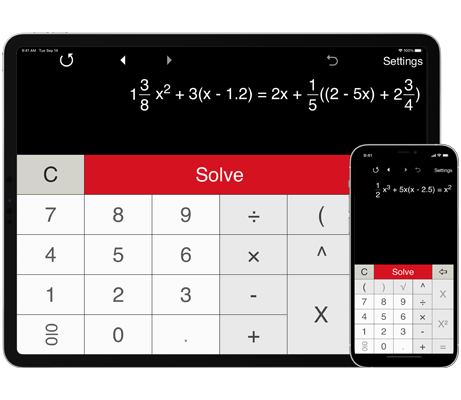# Cubic Equation Solver

Cubic equation solver can handle a wide range of cubic, quadratic and linear equations, including equations with fractions and parentheses.
With the Advanced format, you can input your equation in any form.

## Solving cubic equations

Features:

Cubic Equation Solver helps you quickly solve your homework problems or check your results. It solves cubic, quadratic and linear equations. Just type in any equation you want to solve and Cubic equation solver will show you the result.

With the Advanced format, you can input your equation in any form. It supports equations with fractions and parentheses. Note: the application doesn’t yet support equations with a variable in the denominator.

For cubic equations in the form ax3 + bx2 + cx + d = 0 you can select the Predefined format (in the Settings window). To find the roots of a cubic equation, enter the coefficients ‘a’, ‘b’, ‘c’ and ‘d’ and click 'Solve'. The coefficients ‘a’, ‘b’, ‘c’ and ‘d’ are real numbers, a ≠ 0. Cubic Equation Solver supports positive, negative, or zero values of the coefficients. Note: for a missing term enter zero.

History tape to view recent calculations.

Solves cubic equations using Cardano's formula.

The standard cubic equation (or third degree equation) has the following form: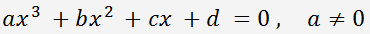#### Solving cubic equations with Cardano's method‎:

Dividing the standard cubic equation by a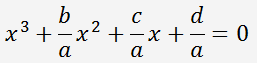and substituting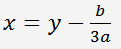we get the following equation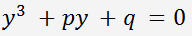,   where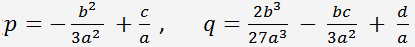The discriminant of the cubic equation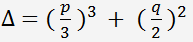.

By Cardano's formulas, the roots of the cubic equation: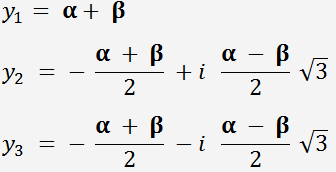where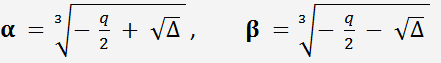For each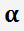you should take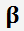, for which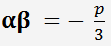If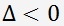,   the equation has three real roots.

If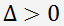,   then one root is real and two are complex conjugates.

If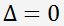,   the equation has two real roots. If p = q = 0, the equation has one real root.